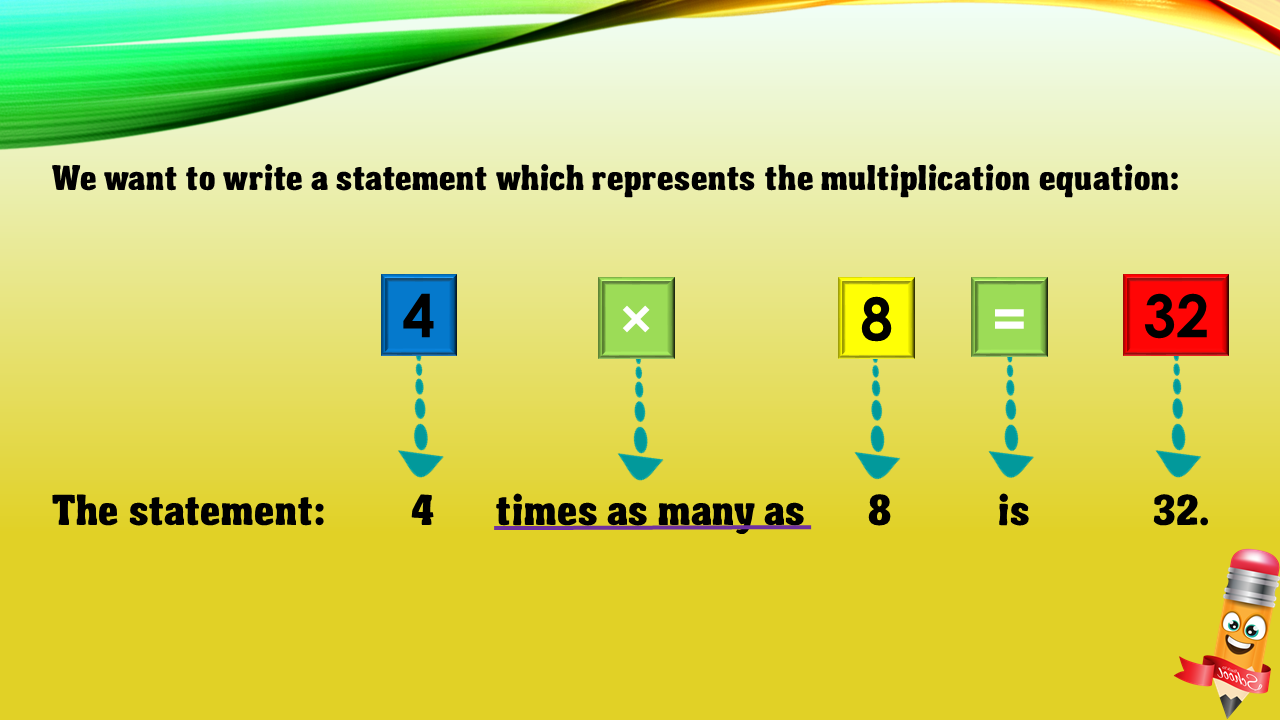1
visibility

Choose one statement which represents the equation:
4 × 8 = 32 ?

• A

4 times as many as 8 is 32.

• B

4 more than 8 is 32.

• C

8 less than 32 is 4.

• D

8 times as many as 32 is 4.